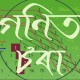## 06 May Mathletics 2013 (Category III) – Assam Academy of Mathematics

(Class IX and X)

Marks: 100

Time: 3 Hours

[Answer the following questions in English or in mother tongue. Two students of the group will discuss the solutions of the problems and write answer in a khata for the group. No third person can help the group to solve the problems]

Marks: 10X10=100

1. Find the smallest number when divided by 3 gives a remainder 1, when divided by 4 gives remainder 2, when divided by 5 gives a remainder 3 and when divided by 6 gives remainder 4.

2. If a>b show that \$\$a^ab^b>a^bb^a.\$\$

3. Two tangents are drawn to a circle at X and Y from a point C. A line AB is drawn tangent to the circle at a point P randomly chosen on the circle’s circumference between X and Y.

If YC=XC=10 units find the perimeter of the triangle ABC.

4. ABC is an isosceles triangle. XY is drawn parallel to the base cutting the sides in X and Y. Show that B, C, X, Y lie in a circle.

5. In a triangle ABC, AB=AC. A transversal intersects AB and AC internally at K and L respectively. It intersects BC produced at M. If KL=2LM, find \$\$frac{KB}{LC}.\$\$

6. If \$\$x^5-x^3+x=a,\$\$ show that \$\$x^6ge 2a-1.\$\$

7. Solve \$\$(4+sqrt{15})^x+(4-sqrt{15})^x=62.\$\$

8. Prove that \$\$n^4+4^n\$\$ is a composite number for all values of n>1.

9. Prove that for every positive integer n,

\$\$1^n+8^n-3^n-6^n\$\$ is divisible by 10.

10. Find the remainder when \$\$(2222)^{5555}\$\$ is divided by 7.

Download this post as PDF (will not include images and mathematical symbols).

##### Tags:
••